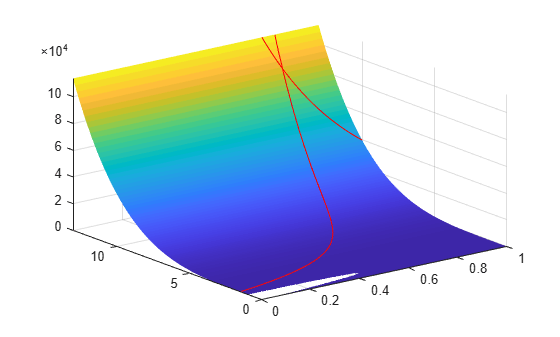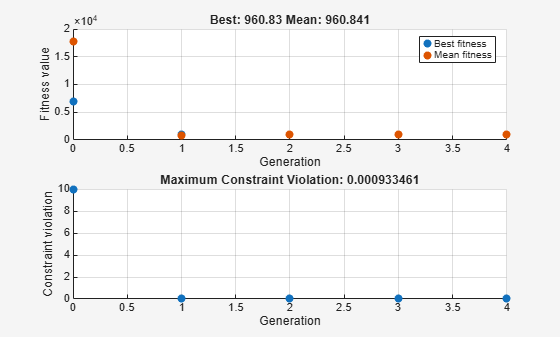# Constrained Minimization Using `ga`, Problem-Based

This example shows how to minimize an objective function, subject to nonlinear inequality constraints and bounds, using `ga` in the problem-based approach. For a solver-based version of this problem, see Constrained Minimization Using the Genetic Algorithm.

### Constrained Minimization Problem

For this problem, the fitness function to minimize is a simple function of 2-D variables `X` and `Y`.

`camxy = @(X,Y)(4 - 2.1.*X.^2 + X.^4./3).*X.^2 + X.*Y + (-4 + 4.*Y.^2).*Y.^2;`

This function is described in Dixon and Szego .

Additionally, the problem has nonlinear constraints and bounds.

``` x*y + x - y + 1.5 <= 0 (nonlinear constraint) 10 - x*y <= 0 (nonlinear constraint) 0 <= x <= 1 (bound) 0 <= y <= 13 (bound) ```

Plot the nonlinear constraint region on a surface plot of the fitness function. The constraints limit the solution to the small region above both red curves.

```x1 = linspace(0,1); y1 = (-x1 - 1.5)./(x1 - 1); y2 = 10./x1; [X,Y] = meshgrid(x1,linspace(0,13)); Z = camxy(X,Y); surf(X,Y,Z,"LineStyle","none") hold on z1 = camxy(x1,y1); z2 = camxy(x1,y2); plot3(x1,y1,z1,'r-',x1,y2,z2,'r-') xlim([0 1]) ylim([0 13]) zlim([0,max(Z,[],"all")]) hold off```### Create Optimization Variables, Problem, and Constraints

To set up this problem, create optimization variables `x` and `y`. Set the bounds when you create the variables.

```x = optimvar("x","LowerBound",0,"UpperBound",1); y = optimvar("y","LowerBound",0,"UpperBound",13);```

Create the objective as an optimization expression.

`cam = camxy(x,y);`

Create an optimization problem with this objective function.

`prob = optimproblem("Objective",cam);`

Create the two nonlinear inequality constraints, and include them in the problem.

```prob.Constraints.cons1 = x*y + x - y + 1.5 <= 0; prob.Constraints.cons2 = 10 - x*y <= 0;```

Review the problem.

`show(prob)`
``` OptimizationProblem : Solve for: x, y minimize : (((((4 - (2.1 .* x.^2)) + (x.^4 ./ 3)) .* x.^2) + (x .* y)) + (((-4) + (4 .* y.^2)) .* y.^2)) subject to cons1: ((((x .* y) + x) - y) + 1.5) <= 0 subject to cons2: (10 - (x .* y)) <= 0 variable bounds: 0 <= x <= 1 0 <= y <= 13 ```

### Solve Problem

Solve the problem, specifying the `ga` solver.

`[sol,fval] = solve(prob,"Solver","ga")`
```Solving problem using ga. Optimization terminated: average change in the fitness value less than options.FunctionTolerance and constraint violation is less than options.ConstraintTolerance. ```
```sol = struct with fields: x: 0.8122 y: 12.3103 ```
```fval = 9.1268e+04 ```

To observe the solver's progress, specify options that select two plot functions. The plot function `gaplotbestf` plots the best objective function value at every iteration, and the plot function `gaplotmaxconstr` plots the maximum constraint violation at every iteration. Set these two plot functions in a cell array. Also, display information about the solver's progress in the Command Window by setting the `Display` option to `'iter'`.

```options = optimoptions(@ga,... 'PlotFcn',{@gaplotbestf,@gaplotmaxconstr},... 'Display','iter');```

Run the solver, including the `options` argument.

`[sol,fval] = solve(prob,"Solver","ga","Options",options)`
```Solving problem using ga. Single objective optimization: 2 Variable(s) 2 Nonlinear inequality constraint(s) Options: CreationFcn: @gacreationuniform CrossoverFcn: @crossoverscattered SelectionFcn: @selectionstochunif MutationFcn: @mutationadaptfeasible Best Max Stall Generation Func-count f(x) Constraint Generations 1 2520 91357.8 0 0 2 4982 91324.1 4.55e-05 0 3 7914 97166.6 0 0 4 16145 91268.4 0.0009997 0 Optimization terminated: average change in the fitness value less than options.FunctionTolerance and constraint violation is less than options.ConstraintTolerance. ``````sol = struct with fields: x: 0.8123 y: 12.3103 ```
```fval = 9.1268e+04 ```

Nonlinear constraints cause `ga` to solve many subproblems at each iteration. As shown in both the plots and the iterative display, the solution process has few iterations. However, the `Func-count` column in the iterative display shows many function evaluations per iteration.

### Unsupported Functions

If your objective or nonlinear constraint functions are not supported (see Supported Operations for Optimization Variables and Expressions), use `fcn2optimexpr` to convert them to a form suitable for the problem-based approach. For example, suppose that instead of the constraint $xy\ge 10$, you have the constraint ${I}_{1}\left(x\right)+{I}_{1}\left(y\right)\ge 10$, where ${I}_{1}\left(x\right)$ is the modified Bessel function `besseli(1,x)`. (The Bessel functions are not supported functions.) Create this constraint using `fcn2optimexpr`. First, create an optimization expression for ${I}_{1}\left(x\right)+{I}_{1}\left(y\right)$.

`bfun = fcn2optimexpr(@(t,u)besseli(1,t) + besseli(1,u),x,y);`

Next, replace the constraint `cons2` with the constraint `bfun >= 10`.

`prob.Constraints.cons2 = bfun >= 10;`

Solve the problem. The solution is different because the constraint region is different.

`[sol2,fval2] = solve(prob,"Solver","ga","Options",options)`
```Solving problem using ga. Single objective optimization: 2 Variable(s) 2 Nonlinear inequality constraint(s) Options: CreationFcn: @gacreationuniform CrossoverFcn: @crossoverscattered SelectionFcn: @selectionstochunif MutationFcn: @mutationadaptfeasible Best Max Stall Generation Func-count f(x) Constraint Generations 1 2512 974.044 0 0 2 4974 960.998 0 0 3 7436 963.12 0 0 4 12001 960.83 0.0009335 0 Optimization terminated: average change in the fitness value less than options.FunctionTolerance and constraint violation is less than options.ConstraintTolerance. ``````sol2 = struct with fields: x: 0.4999 y: 3.9979 ```
```fval2 = 960.8300 ```

### References

 Dixon, L. C. W., and G .P. Szego (eds.). Towards Global Optimisation 2. North-Holland: Elsevier Science Ltd., Amsterdam, 1978.